Function Repository Resource:

# NearEqualPartition

Partition a list into roughly equal lengths

Contributed by: Jon McLoone
 ResourceFunction["NearEqualPartition"][list,n] partitions list into n nonoverlapping sublists of near-equal length.

## Details and Options

When the length of list is divisible by the number of partitions n, all the returned lists will be equal in length. In other cases, ResourceFunction["NearEqualPartition"] maximizes the length of the smallest partition.

## Examples

### Basic Examples (3)

Partition a list into three non-overlapping sublists:

 In:=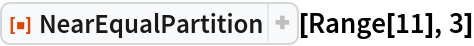Out=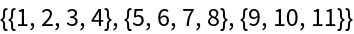When the list cannot be partitioned in equal lengths, the first sublists are the longest:

 In:=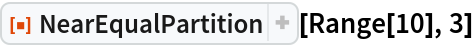Out=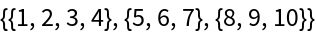When the length of the list is divisible by the partition count, all lists are the same length:

 In:=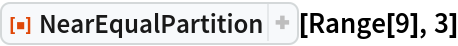Out=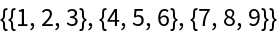### Properties and Relations (2)

No elements of the input are discarded and no extra elements introduced, so for a one-dimensional list the inverse operation is Flatten:

 In:=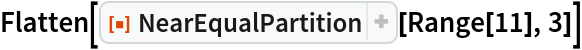Out=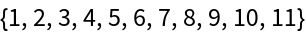The lengths of the sublists should not differ by more than 1:

 In:=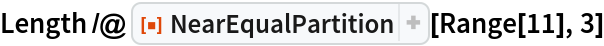Out=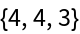Jon McLoone

## Version History

• 1.0.0 – 27 March 2020